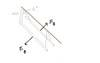# Hard Magnetism Problem

• AznBoi
Since both currents are in the opposite direction, the magnetic force per unit length is also in the opposite direction. The magnitude of the current is 8.3 A.

## Homework Statement

Two long parallel wires, each with a mass per unit length of 40g/m, are supported in a horizontal plane by 6 cm long strings, as shown in the figure below. Each wire carries the same current I, causing the wires to repel each other so that the angle (theta) between the supporting strings is 16 degrees.
a) Are the currents in the same or opposite direction?
b) Determine the magnitude of each current

Figure:## Homework Equations

$$\Sigma F=ma$$

$$B= \frac{\mu_{0}I}{2\pi r}}$$

$$F_{b}=BIl$$

## The Attempt at a Solution

For a) I know that the current must be in the opposite direction.

For b) I drew a Free Body Diagram first and found that $$T_{x}=F_{B} [tex] and these are the same for both wires. However, how do you find the tension if they don't specify the exact weight of each wire? They do tell you that each of the wires have "a mass per unit length of 40g/m" but how do you convert that to weight if you don't know how long the wires are? Thanks! Since you know the force per unit length, all you need is mass per unit length to solve the problem. marcusl said: Since you know the force per unit length, all you need is mass per unit length to solve the problem. Can you elaborate on that please? I still don't get how to solve part b) =/ You don't need the total weight of the wires because you can do the whole problem in terms of unit lengths. You know the mass per unit length so you also know the weight (force downwards due to gravity) per unit length. Since the angle is given, you therefore know the component of force per unit length directed between the wires that exactly balances the magnetic force per unit length. The magnetic force per unit length depends on the current according to the equation you gave, [tex] F_{b}/l=BI$$.
Eliminate B with your equation for B as a function of I, and solve for I.